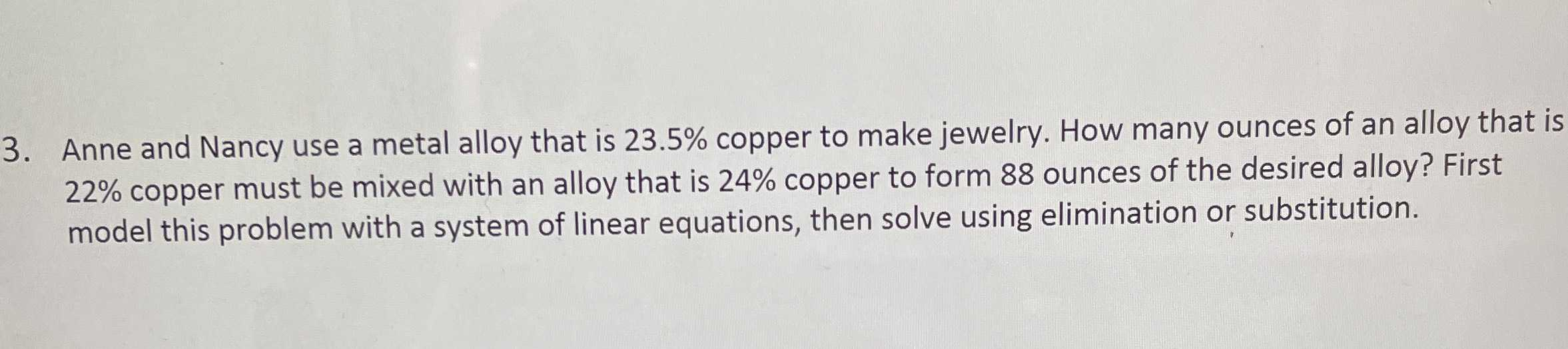### ¿Todavía tienes preguntas de matemáticas?

Pregunte a nuestros tutores expertos
Algebra
Pregunta3. Anne and Nancy use a metal alloy that is $$23.5 \%$$ copper to make jewelry. How many ounces of an alloy that is $$22 \%$$ copper must be mixed with an alloy that is $$24 \%$$ copper to form $$88$$ ounces of the desired alloy? First model this problem with a system of linear equations, then solve using elimination or substitution.

you want the final $$108$$ oz to be $$23.5 \%$$

copper. That means it contains $$25.38$$ oz of copper So, if there are $$x$$ oz of $$21 \%$$ alloy,

$$.21 x + .30 ( 108 - x ) = .235 ( 108 )$$

$$.21 x + 32.4 - .30 x = 25.38$$

$$.09 x = 7.02$$

$$x = 78$$

so, she uses $$78$$ oz of $$21 \%$$ and $$30$$ oz of $$30 \%$$

Solución
View full explanation on CameraMath App.